# Study of harmonic motion of a liquid in a V shaped tube

sumatoken
Homework Statement:
Study of harmonic motion of a liquid in a V shaped tube using the Lagrangian method.
Relevant Equations:
What is the total potential energy of the system? and why is the restoring force considered to be only the one due to hydrostatic pressure?
A V-shaped tube with a cross-section A contains a perfect liquid with mass density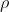and length L plus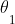and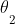the angles between the horizontal plane and the tube arms as shown in the attached figure.

We displace the liquid from its equilibrium position with a distance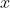and without any initial velocity.

I'm interested in applying the Lagrangian method.

For that, I need to determine both the system's kinetic energy and potential energy.

Kinetic energy is by definition: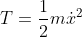As for potential energy I need to know which forces to consider.

I can see three forces applied to the liquid:

- The force due to gravity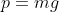with a potential energy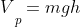- The normal force due to the tube reaction. This force will have a null work therefore no potential energy.

- The force due to hydrostatic pressure on the liquid by the portion displaced of length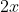with potential energy written in terms of the angles, cross-section A and.

I did some research, and some solutions did not consider the potential energy due to gravity and considered the restoring force to be only the one due to hydrostatic pressure which I do not understand why.

Please feel free to correct me.

Mohammed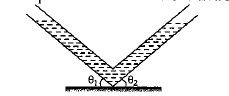Last edited:

Staff Emeritus